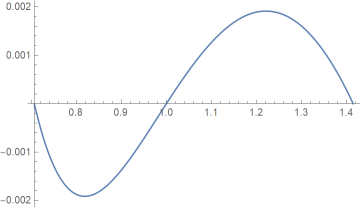# What makes the log10 trick work?

In my post on mentally calculating logarithms, I showed that

log10 x ≈ (x – 1)/(x + 1)

for 1/√10 ≤ x ≤ √10. You could convert this into an approximation for logs in any base by multiplying by the right scaling factor, but why does it work out so simply for base 10?

Define

m(x) = (x – 1)/(x + 1).

Notice that m(1) = 0 and m(1/x) = –m(x), two properties that m shares with logarithms. This is a clue that there’s a connection between m and logs.

Now suppose we want to approximate logb by k m(x) over the interval 1/√ bx ≤ √b. How do we pick k? If we choose

k = 1/(2m(√b))

then our approximation error will be zero at both ends of our interval.

If b = 10, k = 0.9625, i.e. close to 1. That’s why the approximation rule is particularly simple when b = 10. And although it’s good enough to round 0.9625 to 1 for rough calculations, our approximation for logs base 10 would be a little better if we didn’t.

## More bases

In the process of asking why base 10 was special, we came up with a general way of constructing logarithm approximations for any base.

Using the method above we find that

loge ≈ 2.0413 (x – 1)/(x + 1)

over the interval 1/√ex ≤ √e and that

log2 ≈ 2.9142 (x – 1)/(x + 1)

over the interval 1/√2 ≤ x ≤ √2.

These rules are especially convenient if you round 2.0413 down to 2 and round 2.9142 up to 3. These changes are no bigger than rounding 0.9625 up to 1, which we did implicitly in the rule for logs base 10.

## Curiosities

The log base 100 of a number is half the log base 10 of the number. So you could calculate the log of a number base 100 two ways: directly using b = 100 with the method above, or indirectly by setting b = 10 and dividing your result by 2. Do these give you the same answer? Nope! Our scaling is not linear in the (logarithm of) the base.

Well, then which is better? Taking the log base 10 first and dividing by 2 is better. In general, the smaller the base, the more accurate the result.

This seems strange at first, like something for nothing, but realize than when b is smaller, so is the interval we’re working over. You’ve done more work in reducing the range when you use a smaller base, and your reward is that you get a more accurate result. Since 2 is the smallest logarithm base that comes up with any regularity, the approximation for log base 2 is the most accurate of its kind that is likely to come up.

For every base b, we’ve shown that the approximation error is zero at 1/√b, 1, and √b. What we haven’t shown is that we have what’s known as “equal ripple” error. For example, here’s a plot of the error for our rule for approximating log base 2.The error goes exactly as far negative on the left of 1 as it goes positive on the right of 1. The minimum is -0.00191483 and the maximum is 0.00191483. This follows from the property

m(1/x) = –m(x)

mentioned above. The location of the minimum (signed) error is the reciprocal location of the maximum error, and the value of that minimum is the negative of the maximum.

Update: The approximations from this post and several similar posts are all consolidated here.

## One thought on “What makes the log10 trick work?”

1. You can also get a quadratic rational function m with m(1)=0 and m(1/x)=-m(x) as (x-1)(x+1)/(d(x^2+1) + ex) for any d,e. (And a calculation shows that these are all the solutions if you want a quadratic over a quadratic that isn’t just a multiple of (x-1)/(x+1). To avoid that in the preceding form you just need e different from 2d and -2d.) Of course, now it’s harder to do in your head, but I’m curious if one optimizes for the choice of d,e how much better the error gets. When I tried d=1.5 and e=1 the error was pretty decent, but I didn’t do the complete optimization.